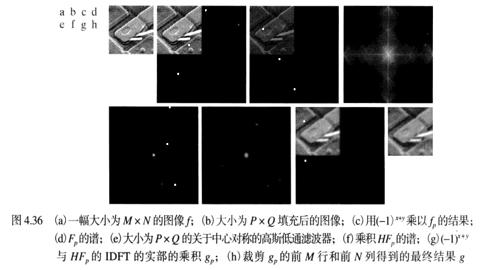傅里叶变换频域中的滤波基础

1. 将 M * N 大小的图像扩展到 2M * 2N, 多余像素以 0 填充
2. ${\left(-1\right)}^{M+N}$ 乘以输入图像进行中心变换
3. 计算图像的 DFT, 即 $F\left(u,v\right)$
4. 用滤波器函数 $H\left(u,v\right)$ 乘以 $F\left(u,v\right)$
5. 计算 4 中结果的反 DFT
6. 得到 5 中结果的实部
7. ${\left(-1\right)}^{M+N}$ 乘以 6 中的结果
8. 提取 7 中结果左上象限 M * N 大小的区域$F\left(u,v\right)$ 的中心部分为低频信号, 边缘部分为高频信号. 高频信号保存了图像的细节. $H\left(u,v\right)$ 也被称为滤波器. 输出图像的傅里叶变换为:

$G\left(u,v\right)=F\left(u,v\right)H\left(u,v\right)$

$H$$F$ 的相乘涉及二维函数, 并在逐元素的基础上定义. 即: $H$ 的第一个元素乘以 $F$ 的第一个元素, $H$ 的第二个元素乘以 $F$ 的第二个元素, 以此类推.

相关代码

``````# 图像的傅里叶变换与反变换
import numpy as np
import scipy.misc
import PIL.Image
import matplotlib.pyplot as plt

im = PIL.Image.open('/img/jp.jpg')
im = im.convert('L')
im_mat = scipy.misc.fromimage(im)
rows, cols = im_mat.shape

# 扩展 M * N 图像到 2M * 2N
im_mat_ext = np.zeros((rows * 2, cols * 2))
for i in range(rows):
for j in range(cols):
im_mat_ext[i][j] = im_mat[i][j]

# 傅里叶变换
im_mat_fu = np.fft.fft2(im_mat_ext)
# 将低频信号移植中间, 等效于在时域上对 f(x, y) 乘以 (-1)^(m + n)
im_mat_fu = np.fft.fftshift(im_mat_fu)

# 显示原图
plt.subplot(121)
plt.imshow(im_mat, 'gray')
plt.title('original')
plt.subplot(122)
# 在显示频率谱之前, 对频率谱取实部并进行对数变换
plt.imshow(np.log(np.abs(im_mat_fu)), 'gray')
plt.title('fourier')
plt.show()

# 傅里叶反变换
im_converted_mat = np.fft.ifft2(np.fft.ifftshift(im_mat_fu))
# 得到傅里叶反变换结果的实部
im_converted_mat = np.abs(im_converted_mat)
# 提取左上象限
im_converted_mat = im_converted_mat[0:rows, 0:cols]
# 显示图像
im_converted = PIL.Image.fromarray(im_converted_mat)
im_converted.show()
``````